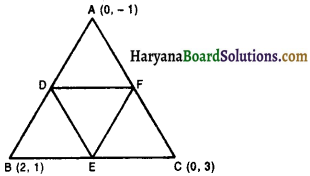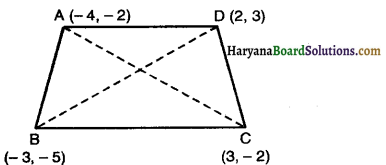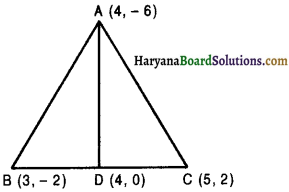# HBSE 10th Class Maths Solutions Chapter 7 Coordinate Geometry Ex 7.3

Haryana State Board HBSE 10th Class Maths Solutions Chapter 7 Coordinate Geometry Ex 7.3 Textbook Exercise Questions and Answers.

## Haryana Board 10th Class Maths Solutions Chapter 7 Coordinate Geometry Ex 7.3

Question 1.
Find the area of the triangle whose vertices are :
(i) (2, 3), (- 1, 0), (2, – 4)
(ii) (- 5, – 1), (3, – 5), (5, 2)
Solution:
(i) Let A (2, 3), B (- 1, 0) and C(2, – 4) be the vertices of the given triangle.
Here, x = 2, y = 3, x = -1, y = 0, x = 2, y = – 4
∴ Area of ∆ABC = $$\frac{1}{2}$$ [x1(y2 – y3) + x2(y3 – y1) + x3(y1 – y2)]
= $$\frac{1}{2}$$ [2(0 + 4)+ (- 1)(- 4 – 3) + 2(3 – 0)]
= $$\frac{1}{2}$$ [8 + 7 + 6]
= $$\frac{1}{2}$$ × 21 = 10.52
Hence, Area of ∆ABC = 10.5 square units.

(ii) Let A(- 5, – 1), B(3, – 5) and C(5, 2) be the vertices of given triangle.
Here x1 = – 5, y1 = – 1, x2 = 3, y2 = 5, x3 = 5, y3 = 2
∴ Area of ∆ABC = $$\frac{1}{2}$$ [x1(y2 – y3) + x2(y3 – y1) + x3(y1 – y2)]
= $$\frac{1}{2}$$ [- 5(- 5 – 2) + 3(2 + 1) + 5 (- 1+ 5)]
= $$\frac{1}{2}$$ [- 5 × – 7 + 3 × 3 + 5 × 4]
= $$\frac{1}{2}$$ (35 + 9 + 20)
= $$\frac{1}{2}$$ (64) = 32
Hence, Area of ∆ABC = 32 square units.Question 2.
In each of the following find the value of ‘k’, for which the points are collinear.
(i) (7, – 2), (5, 1), (3, k)
(ii) (8, 1), (k, – 4), (2, – 5)
Solution :
(i) Let the given points be A(7, – 2), B(5, 1) and C(3, k)
Here, x1 = 7, y1 = – 2, x2 = 5, y2 = 1, x3 = 3, y3 = k
Since, the given points are collinear.
Area of ∆ABC = 0
⇒ $$\frac{1}{2}$$ [x1(y2 – y3) + x2(y3 – y1) + x3(y1 – y2)] = 0
⇒ $$\frac{1}{2}$$ [7(1 – k) + 5(k+ 2) + 3(- 2 – 1)] = 0
⇒ $$\frac{1}{2}$$ [7 – 7k + 5k + 10 + 3 × (- 3)] = 0
⇒ $$\frac{1}{2}$$ (- 2k + 17 – 9)= 0
⇒ $$\frac{1}{2}$$ (-2k + 8) = 0
⇒ – 2k + 8 = 0
⇒ – 2k = – 8
⇒ k = $$\frac{-8}{-2}$$
⇒ k = 4
⇒ Hence, k = 4.

(ii) Let the given points be A(8, 1), B(k, – 4) and C(2, – 5)
Here, x1 = 8, y1 = 1, x2 = k, y2 = – 4, x3 = 2, y3 = – 5
Since, the given points are collinear.
Area of ∆ABC = 0
⇒ $$\frac{1}{2}$$ [x1(y2 – y3) + x2(y3 – y1) + x3(y1 – y2)] = 0
⇒ $$\frac{1}{2}$$ [8(- 4 + 5) + k(- 5 – 1) + 2(1 + 4)] = 0
⇒ $$\frac{1}{2}$$ [8 × 1 + k × (- 6) + 2 × 5] = 0
⇒ $$\frac{1}{2}$$ [8 – 6k + 10] = 0
⇒ (- 6k + 18) = 0
⇒ – 6k + 18 = 0
⇒ 6k = 18
⇒ k = $$\frac{18}{6}$$
⇒ k = 3
Hence, k = 3.Question 3.
Find the area of the triangle formed by the joining the mid-points of the sides of the triangle whose vertices are (0, -1), (2, 1) and (0, 3). Find the ratio of this area to the area of the given triangle.
Solution :
Let A (0, – 1), B (2, 1) and C (0, 3) be the vertices of given triangle.
Let D, E and F are the mid points of sides of the triangle AB, BC and CA respectively. Then,
The co-ordinates of D are $$\left(\frac{0+2}{2}, \frac{-1+1}{2}\right)$$ i.e., (1, 0)
The co-ordinates of E are $$\left(\frac{2+0}{2}, \frac{1+3}{2}\right)$$ i.e., (1, 2)
The co-ordinates of F are $$\left(\frac{0+0}{2}, \frac{-1+3}{2}\right)$$ i.e., (0, 1)Thus, co-ordinates of the mid-points are D(1, 0), E(1, 2), F(0, 1)
∴ Area of ∆DEF = $$\frac{1}{2}$$ [x1(y2 – y3) + x2(y3 – y1) + x3(y1 – y2)]
= $$\frac{1}{2}$$ [1(2 – 1) + 1(1 – 0) + 0(0 – 2)]
= $$\frac{1}{2}$$ (1 × 1 + 1 × 1 + 0)
= $$\frac{1}{2}$$ (1 + 1)
= $$\frac{1}{2}$$ × 2
= 1 square unit.
Now, Area of ∆ABC = $$\frac{1}{2}$$ [x1(y2 – y3) + x2(y3 – y1) + x3(y1 – y2)]
= $$\frac{1}{2}$$ [0(1 – 3) + 2(3 + 1) + 0(- 1- 1)]
= $$\frac{1}{2}$$ [0(- 2) + 2 × 4 + 0 (- 2)]
= $$\frac{1}{2}$$ (0 + 8 + 0)
= $$\frac{1}{2}$$ × 8
= 4 square units.
Area of ∆DEF : Area of ∆ABC = 1 : 4
Hence, area of ∆DEF = 1 square unit and
Area of ∆DEF : Area of ∆ABC =1 : 4.Question 4.
Find the area of the quadrilateral whose vertices, taken in order, are(- 4, – 2), (- 3, – 5), (3, – 2) and (2, 3).
Solution :
Let A(- 4, – 2), B(- 3, – 5), C(3, – 2) and D(2, 3) be the vertices of a quadrilateral. Join A to C.
Area of quadrilateral ABCD = Area(∆ABC) + Area(∆ACD)
Now, Area of ∆ABC = $$\frac{1}{2}$$ [x1(y2 – y3) + x2(y3 – y1) + x3(y1 – y2)]
= $$\frac{1}{2}$$ [- 4(- 5 + 2) + (- 3) (- 2 + 2) + 3 (- 2 + 5)]
= $$\frac{1}{2}$$ [- 4 × – 3 – 3 × 0 + 3 × 3]
= $$\frac{1}{2}$$ (12 – 0 + 9)= $$\frac{21}{2}$$ square units.
and, Area of ∆ACD = $$\frac{1}{2}$$ [x1(y2 – y3) + x2(y3 – y1) + x3(y1 – y2)]
= $$\frac{1}{2}$$ [- 4(- 2 – 3)+ 3(3 + 2) + 2(- 2 + 2)]
= $$\frac{1}{2}$$ [- 4 × (- 5) + 3 × 5 + 2 × 0]
= $$\frac{1}{2}$$ (20 + 15 + 0)
= $$\frac{1}{2}$$ × 35
= $$\frac{35}{2}$$
Hence, Area of quadrilateral ABCD = $$\frac{21}{2}$$ + $$\frac{35}{2}$$
= $$\frac{56}{2}$$ = 28 square units.Question 5.
You have studied in class IX, that a median of a triangle divides it into two triangles of equal areas.Verify this result for ∆ABC whose vertices are A(4, – 6), B(3, – 2) and C(5, 2).
Solution :
∴ D is the mid point of BC. Then co-ordinates of D are $$\left(\frac{3+5}{2}, \frac{-2+2}{2}\right)$$ i.e., (4, 0)Now Area of ∆ABD = $$\frac{1}{2}$$ [x1(y2 – y3) + x2(y3 – y1) + x3(y1 – y2)]
= $$\frac{1}{2}$$ [4(- 2 – 0) + 3(0 + 6) + 4(- 6 + 2)]
= $$\frac{1}{2}$$ [4 × (- 2) + 3 × 6 + 4 × (- 4)]
= $$\frac{1}{2}$$ [- 8 + 18 – 16]
= $$\frac{1}{2}$$ (- 24 + 18)
= $$\frac{1}{2}$$ × – 6 = – 3
Since, area cannot be negative, we will take numerical value of – 3 i.e. 3 square units and
area of ∆ADC = $$\frac{1}{2}$$ [x1(y2 – y3) + x2(y3 – y1) + x3(y1 – y2)]
= $$\frac{1}{2}$$ [4(0 – 2) + 4(2 + 6) + 5(- 6 – 0)]
= $$\frac{1}{2}$$ [4 × (- 2) + 4 × 8 + 5 (- 6)]
= $$\frac{1}{2}$$ (- 8 + 32 – 30)
= $$\frac{1}{2}$$ (- 6)
= – 3 i.e., 3 square units.
Hence Area of ∆ABD = Area of ∆ADC.